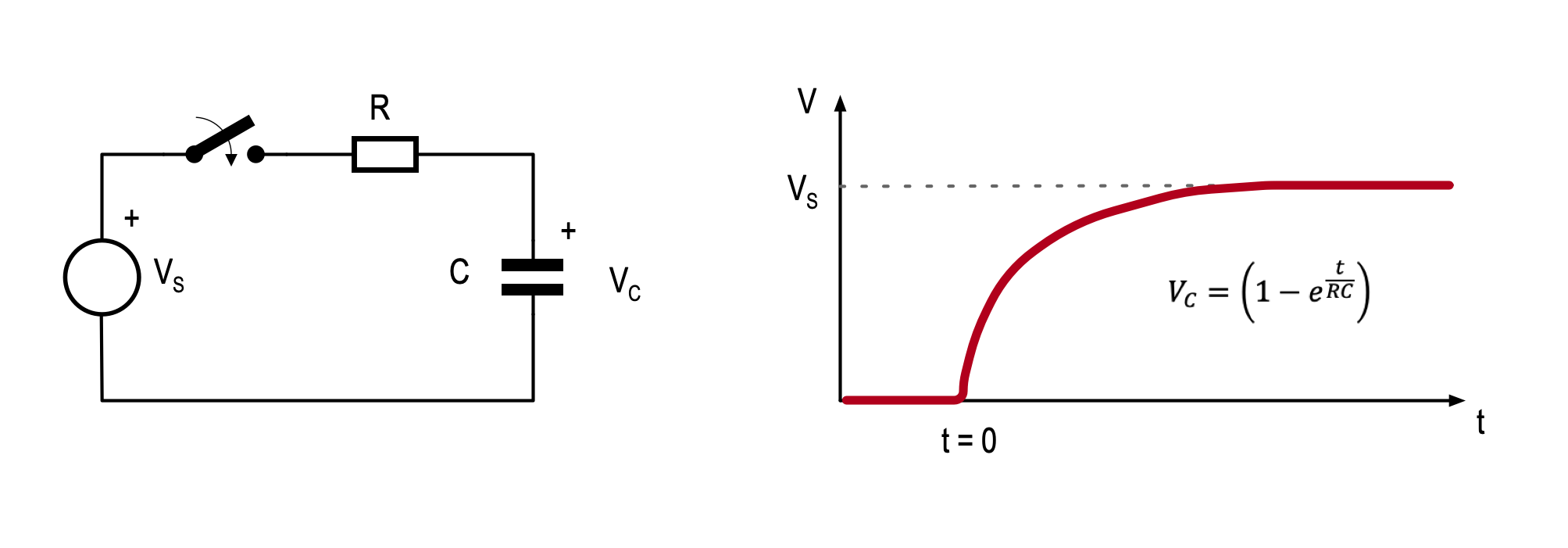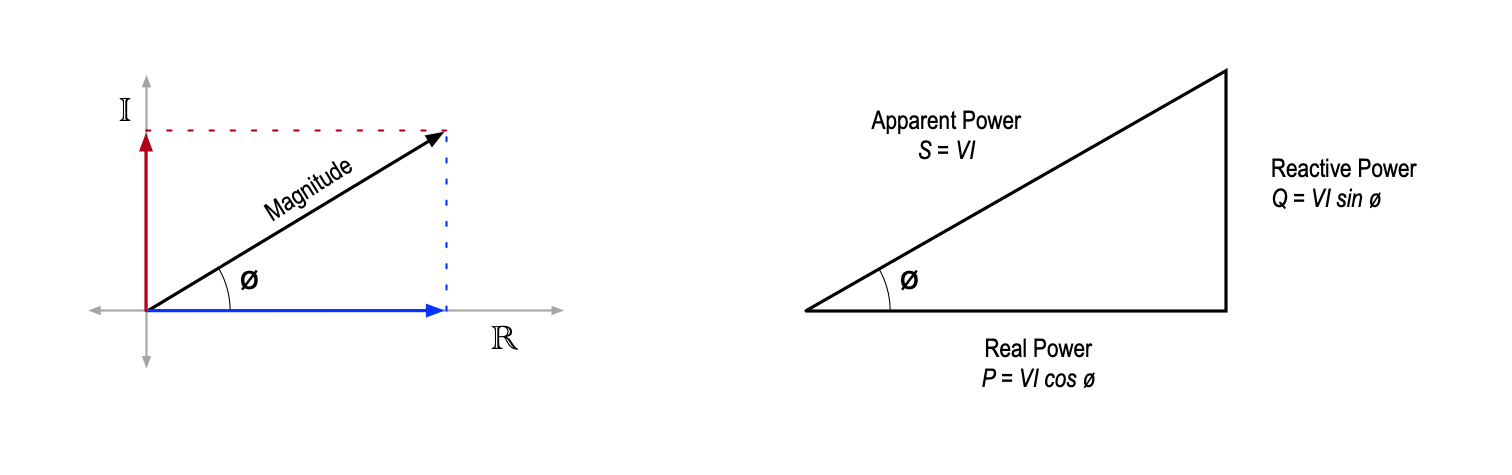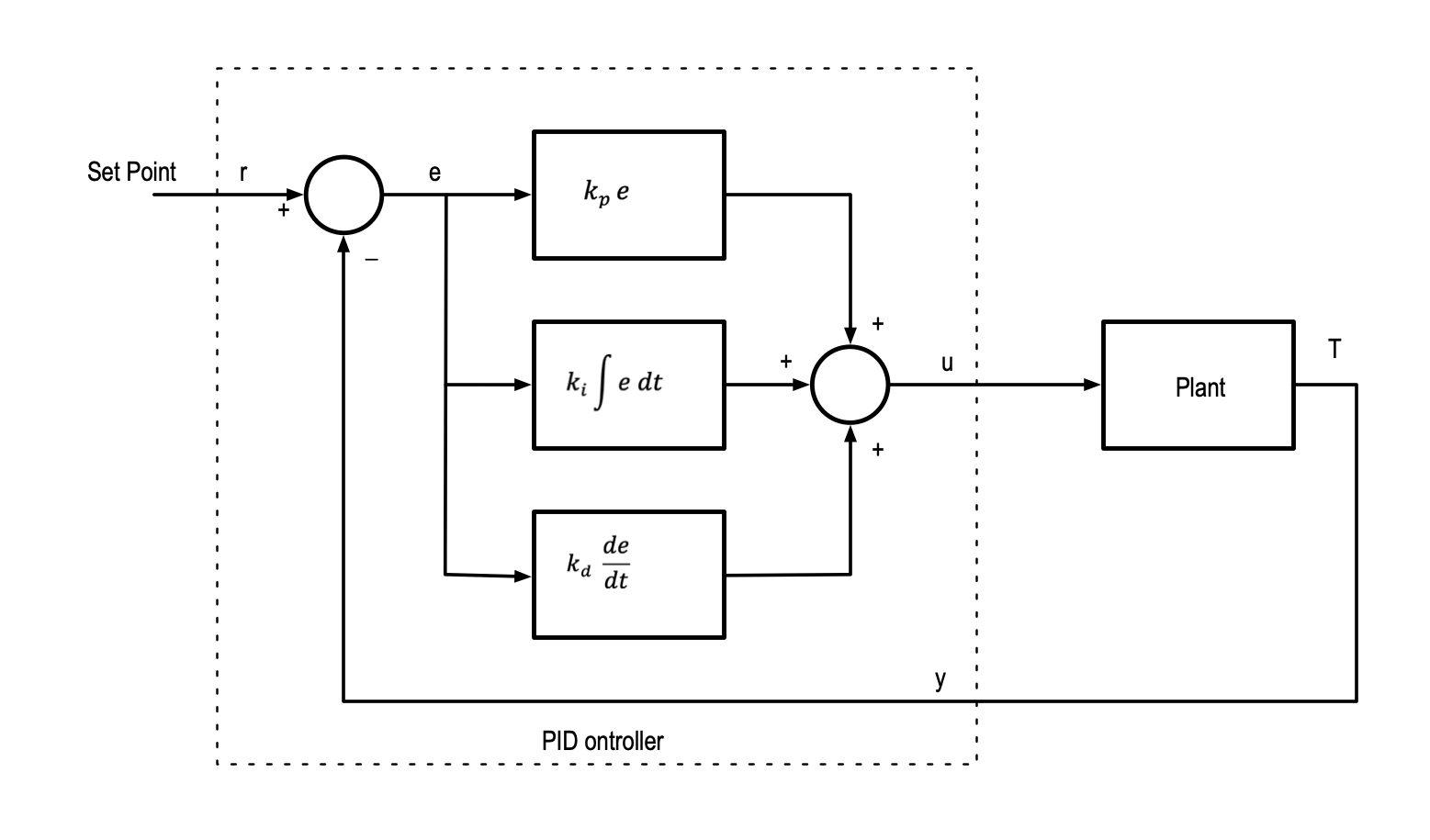# How Much Maths Do You Need?Written by

How much mathematics do you actually need to know to design electronics? Traditional electrical engineering degree courses are maths-heavy, but I would argue that you don’t need to understand Maxwell’s equations to design innovative and effective circuits.

The great thing about electronics is that you can start with minimal maths and be very productive. As you encounter design challenges, or problems that require a bit more maths, you can learn that as you go. For many of us (and I am definitely in this category) learning mathematics for its own sake is not that exciting – however learning a bit of maths as a tool to solve a problem or understand a circuit is a different matter altogether.

I have presented here a kind of progression of mathematical concepts that I think are useful in the field of electronics, in a kind of order of importance. Of course, what’s important to you will depend on the problem you are trying to solve.

I would argue that only a basic level of maths proficiency is necessary to get started, but this need only be some simple high-school algebra and the ability to use a pocket calculator.  Indeed, if you are focussed on digital circuits and microcontrollers this may be all you ever need.

A good example of what can be achieved with just a bit of algebra is the analysis of the relatively complex Howland Current Source shown in Figure 1. There is no more involved than addition, subtraction, multiplication & addition, yet we can derive a simple expression for Iout as shown.Figure 1This Howland current source can source or sink a current at its output node proportional to the voltage at its input node. Analysing this relatively complex circuit requires basic maths operations and some algebraic manipulation. You can go a long way in electronics with a very little maths.

If we need to get a bit more in-depth, particularly with discrete circuits, we could add a little knowledge of exponentials and logarithms to our mathematical toolbox. This is not too much of a stretch and will prove useful when describing quantities that extend over many orders of magnitude and in describing the response of capacitors and inductors to a step change. Figure 2 shows that the equation for the voltage on a simple RC circuit is exponential in nature.Figure 2Many natural phenomena, including many in electronics, follow an exponential or logarithmic pattern. In this example the voltage across a capacitor at a given point in time as it is charged from a fixed voltage source via a resistor is given by the an equation involving an exponential. The symbol e represents Euler’s number (approximately 2.7183).

Many other useful relationships follow exponential (or conversely, logarithmic) relationships, including the Ebers-Moll model which describes the large-signal relationship between BJT base-emitter voltage and collector current.

Trigonometry & Vectors. As soon as we move from DC circuits to AC circuits, it does help to know a bit of trig and understand simple vectors. This is because in AC circuits we are concerned with both the magnitude and phase of voltages and currents. We tend to use vectors to describe these quantities since we can express the magnitude by the vector length and the phase by the angle of the vector to horizontal.

A really good example of this is the calculation power in a reactive circuit as shown in Figure 3. The real power (that which will be dissipated in the circuit) is related to the magnitudes of the voltage and the cosine of the phase angle between them. Apparent power is the simple product of the magnitudes of voltage and current.Figure 3When we consider AC circuits we are often concerned about the relative phase of signals as well as their magnitude. We can depict a sinusoidal waveform as a vector or phasor and shown on the left. The vector has a magnitude represented by its length and a phase represented by the angle form the horizontal. Where we have an AC voltage and current in a reactive circuit, we can use trigonometry to calculate such things as the real power (which will be dissipated in the circuit).  We could equally use complex numbers to represent the vector if we consider its projections onto the real and imaginary axes.

The left hand side of Figure 3 shows that we can describe a vector as an arrow with a length corresponding to its magnitude and an angle from horizontal corresponding to its phase. We can equally describe the same vector by its projection on the horizontal and vertical axes as shown in red and blue.

The diagram on the left of Figure 3, and the name “real power” hints at yet another piece of mathematics that is extremely useful in analysis of AC circuits. This is the application of complex numbers.

Just as real numbers can be expressed as a point on a number line extending to infinity in each direction, complex numbers can be expressed as a point on a plane extending to infinity in all directions. Complex numbers provide us with an alternative way to describe magnitude and phase that greatly simplifies some calculations

Finally, no discussion of mathematics for electronics would be complete without some mention of calculus. Calculus is a great mathematical tool for understanding continuous phenomena (such as how voltages and currents change in analogue circuits. Many people are intimidated by calculus (unnecessarily in my opinion) but I would suggest that you don’t need to be an expert at calculating derivatives or integrals to understand and apply the concepts at a broad level.

Take for example the classic PID control loop as shown in Figure 4. Here there is an integral term and a differential. The integral term is proportional to the running sum of the error and the differential term is proportional to the rate of change of the error. These are not difficult concepts to grasp – and once you have done so it is a pretty easy leap to the maths.Figure 4You don’t need to be an expert in calculus to design electronics, but a high-level appreciation of the concepts of derivatives and integrals can be very helpful at times. This diagram represents the ubiquitous PID (Proportional – Integral – Derivative) controller. The integral term is concerned with a running total of the error term and the derivative term is concerned with the rate of change of the error term.

Of course, there is a lot more maths that is useful for electronics, such as matrices and probability, to name just two, and even more if you want to get into the firmware side. My point is really to say that you don’t need a lot of maths to be effective as an electronics designer. At the same time, you should not be intimidated by more advanced mathematics topics and be open to learning more as and when it is required. You will most likely find that if you are motivated to solve a particular problem, or better understand some circuit, the maths will come much more easily than it will if you were learning it for its own sake.

References

“Working with Exponents and Logarithms.” Accessed December 16, 2022. https://www.mathsisfun.com/algebra/exponents-logarithms.html.

“Phasor Introduction and Demo.” Accessed December 16, 2022. https://lpsa.swarthmore.edu/BackGround/phasor/phasor.html.

 Keep up-to-date with our FREE Weekly Newsletter! Don't miss out on upcoming issues of Circuit Cellar. Subscribe to Circuit Cellar Magazine Note: We’ve made the May 2020 issue of Circuit Cellar available as a free sample issue. In it, you’ll find a rich variety of the kinds of articles and information that exemplify a typical issue of the current magazine. Would you like to write for Circuit Cellar? We are always accepting articles/posts from the technical community. Get in touch with us and let's discuss your ideas.